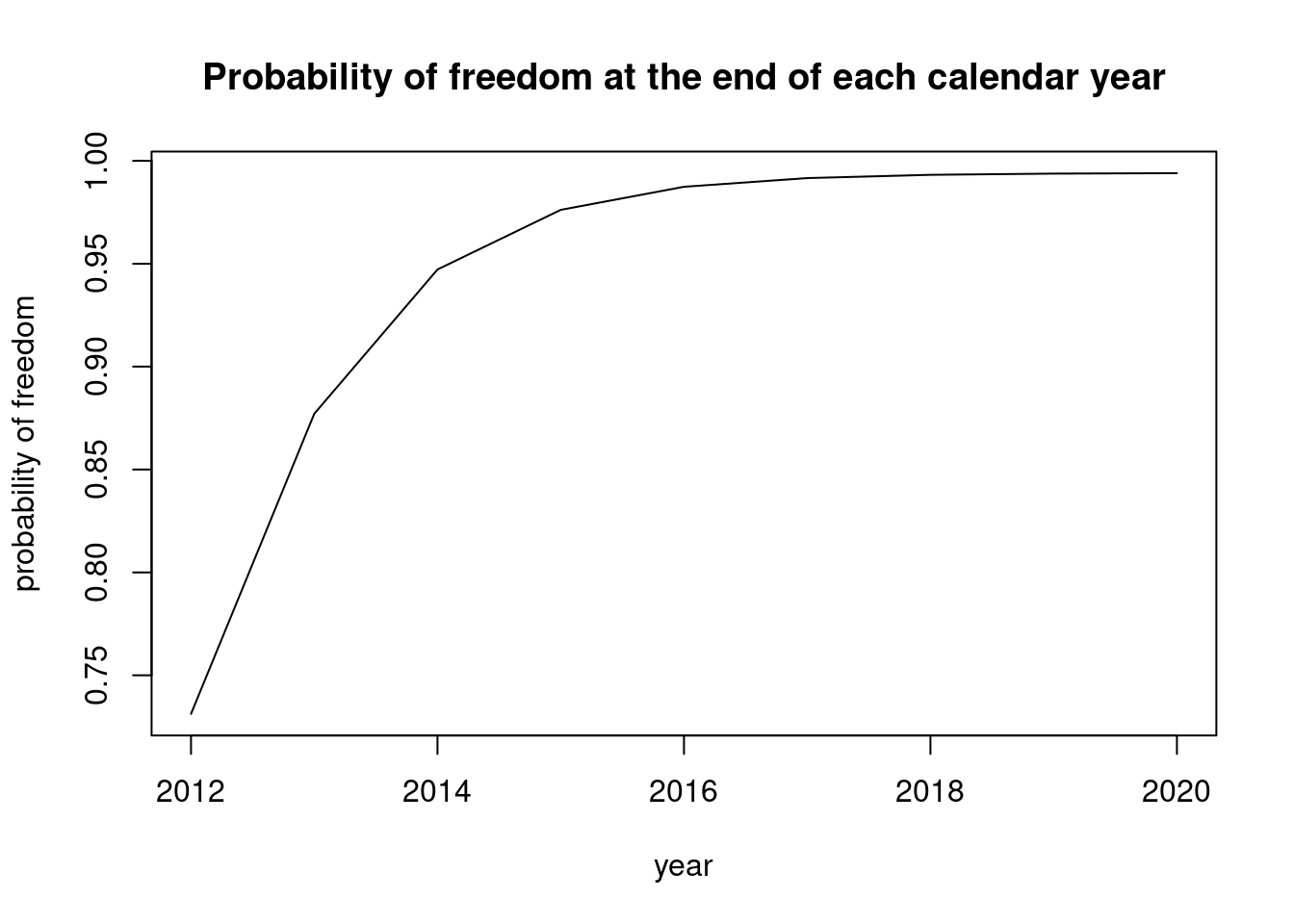# Simple freedom calculation

## Scenario

You have a population of 10000 herds of which 500 are tested per year using a test that has a herd sensitivity (HSe) of 20%; all of these tests were negative. You need to calculate the annual surveillance system sensitivity (SysSe) and the probability that the disease has a lower than 1% prevalence (dp) in the population of herd over time given a prior assumption that the probability of the disease being absent from the population is 50% (prior_pr). You also assume that the annual cumulative probability of introduction to the population is 1% (prob_intro). This programme was ongoing from 2012 to 2020.

## Calculate system sensitivity

library(freedom)

Hse <- rep(0.2, 500)
dp <- rep(0.01, 500)
SysSe <- sysse(dp, Hse)

## Temporal discounting

The surveillance system has a sensitivity of detecting the disease at a prevalence of greater than of equal to 0.01 is 0.6324887 for 1 year. We can then use this to calculate the probability of freedom of disease over time.

prior_pr <- 0.5
prob_intro <- 0.01

pr_free <- data.frame(year = 2012:2020,
prior_fr = NA,
post_fr = NA,
stringsAsFactors = FALSE)
## At the beginning of the first year the probability of freedom is just
## the prior.

pr_free$prior_fr <- prior_pr pr_free$post_fr <- post_fr(pr_free$prior_fr, SysSe) ## Then we use the temporal discouting proceedure to calculate the subsequent ## years: for (i in seq(2, nrow(pr_free))) { pr_free$prior_fr[i] <- prior_fr(pr_free$post_fr[i - 1], prob_intro) pr_free$post_fr[i] <- post_fr(pr_free$prior_fr[i], SysSe) } ## Plot results Now we have the prior and posterior probability of freedom in the population for each of the 8 years of surveillance: pr_free ## year prior_fr post_fr ## 1 2012 0.5000000 0.7312554 ## 2 2013 0.7239429 0.8770845 ## 3 2014 0.8683136 0.9472066 ## 4 2015 0.9377345 0.9761786 ## 5 2016 0.9664168 0.9873900 ## 6 2017 0.9775161 0.9916177 ## 7 2018 0.9817015 0.9931964 ## 8 2019 0.9832644 0.9937837 ## 9 2020 0.9838458 0.9940019 plot(x = pr_free$year,
y = pr_free\$post_fr,
type = "l",
xlab = "year",
ylab = "probability of freedom",
main = "Probability of freedom at the end of each calendar year")